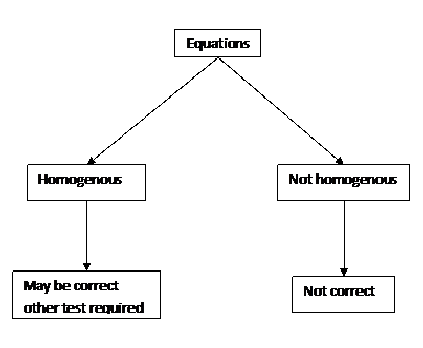Custom Search
If you want to discuss more on this or other issues related to Physics, feel free to leave a message on my Facebook page.

## Saturday, February 13, 2010

### Homogeneity of equations

As we have seen in the chapter on derived units some quantities have units that is composed of two or more base units.

The physics students will meet with different equations. Now some of these equations are correct while some of them are not.

The test to check whether an equation is correct is through the homogeneity test as shown in fig 1 below.Fig 1

So the question is how to check whether an equation is homogenous?

Example 1

Check whether the following equation is homogenous?

P =hdg

Where p is pressure. h is height, d is density and g is the acceleration due to gravity.

As you can see the equation has two sides. The left-hand side and the right hand side.

Now you  have to check that both side of the equation has the same unit.

Pressure = Force / Surface area

Unit of pressure = Unit of force/ unit of area

= kg m s-2 / m2

= kg m-1 s-2

unit of hdg = unit of h* unit of d* unit of g

= m * kg m-3 * m s-2

= kg m-1 s-2

Since both side of the equation has the same unit then the equation is homogenous.

Please note that if their is a dimensionless constant in the equation like k, 1/2 or something like that then the dimensionless constant, by definition, has no unit and as a result will not be involved when the test for homogeneity is performed.

Example 2

Check whether the following equation is homogenous.

v2 = u2 + 2as

In this case the equation is composed of three groups of quantities: v2 , u2 and 2as. You should check that the three groups of quantities have the same unit.

If the three group have the same unit the equation is homogenous otherwise it is not homogenous.

Note: Please see chan’s comment below for a more detailed  answer to example 2

1.I understood the first one completely seems simple enough but on the second I could only get as far as:

v2 = u2 + 2as

(ms-1)2 = (ms-1)2 + 2( m/s2 * m)

Think you can help?
Thanks in advance, great page by the way !

1.v^2= u^2+2as. 2 is a constant so its not counted it.
(ms^-1)^2= (ms^-1)^2 + (ms^-2)(m)
(m^2s^-2) = m^2s^-2 + m^2s^-2. They are all the same, right? Hope I helped you.

2.is the unit of s, m. I believe it's s.

3.s is displacement, not time. So it is in metres.

4.is the equation v=wr in circular motion homogeneous? as w is in rads-1, r is in m and v is in ms-1
Thanks